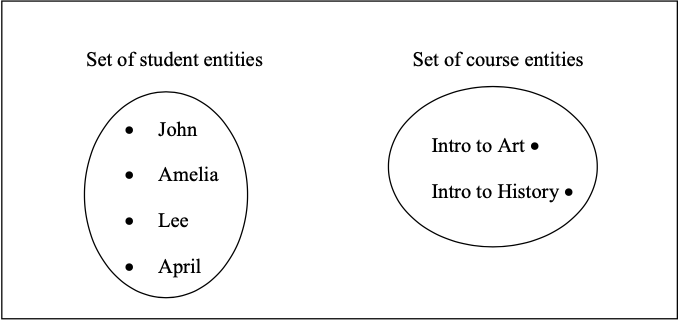# 10.2.1.4: Introduction

•• Professor (Applied Computer Science) at University of Winnipeg

In the entity relationship approach to modeling we analyze system requirements and classify our knowledge in terms of entities, relationships, and attributes.

Entities

Entities are the things we decide to keep track of. For example, if one considers a system to support an educational environment, one is likely to decide that we need to keep track of students, instructors, courses, etc. Typically entities are the people, places, things, and events that we need to remember something about.

Suppose we know of four student entities and two course entities. For example, consider four students (say John, Amelia, Lee, and April) and two courses (Introduction to Art and Introduction to History). We can illustrate these in a number of ways:

As tables of information:Figure $$\PageIndex{1}$$: Entities shown as rows in table.

As sets of entities:Figure $$\PageIndex{2}$$: Entities shown as sets.

Relationships

Entities can be related to one another and so we use relationships to describe how entities relate to one another. Continuing with our educational example we know that students enroll in courses, and so this is one of the relationships we should know about. Suppose we have the two courses and four students listed previously. Suppose also that

• John and Amelia are enrolled in Introduction to Art
• John and Lee are enrolled in Introduction to History
• April is not enrolled in any course.

Below, we depict four instances of the enroll-in relationship by drawing a line from a student to a course. Each relationship pairs one student with one course.Figure $$\PageIndex{3}$$: Relationships shown as lines connecting entities.

Attributes

Entities and relationships have characteristics that describe them. For instance, the students in our example are described by the values for their name, id number, and phone number. As we look back we can see there is a student named John whose id number is 184 and his phone number is 283-4984.

Courses are shown with a course title, a course number, and to belong to a department. There is a course numbered 661 that is offered by the Art department and it is titled Introduction to Art.

If we consider the enroll-in relationship we know there is a date when the student enrolled in the course and a final grade that was awarded to the student when the course was completed. For instance, we could have that John enrolled in Introduction to Art on July 1, 2010 and was awarded an A+ on completion of that course.

These characteristics that serve to describe entities and relationships are called attributes. We will be examining attributes in some detail. As we will see some attributes, such as student number, serve to distinguish one instance from another - each student has a student number distinct from any other student. Other attributes we consider to be purely descriptive, such as the name of a student - many students could have the same name.

Notation

There are many notations in use today that illustrate database designs. In this text, as is done in many database textbooks, the Peter Chen notation is used; other popular notations include IDEF1X, IE and UML. There are many similarities, and so once you master the Peter Chen notation it is not difficult to adapt to a different notation.

The following is an example of an ERD drawn using the Peter Chen notation. Note the following:

• Entity types are represented using rectangular shapes.
• Relationship types are shown with a diamond shape. Lines connect the relationship type to its related entity types with cardinality symbols (m and n).
• Attributes are shown as ovals with a line connecting it to the pertinent entity type or relationship type.Figure $$\PageIndex{4}$$: An ERD in Chen notation.

The various symbols we use with the Peter Chen notation:Figure $$\PageIndex{5}$$: Symbols used in the Chen notation.